###### Indexed In
• Genamics JournalSeek
• JournalTOCs
• CiteFactor
• RefSeek
• Hamdard University
• EBSCO A-Z
• OCLC- WorldCat
• Publons

Chicago, USA

### 5th International Conference on Aerospace, Defense and Mechanical Engineering

Amsterdam, Netherlands

### International Conference on Mechanical and Aerospace Engineering

Frankfurt, Germany

### 10th International Conference and Exhibition on Mechanical & Aerospace Engineering

###### Journal Flyer###### Open Access Journals

Research - (2019) Volume 8, Issue 2

##### Interface Liquid-Solid: Global Bifurcation Multiparameter of Flow Water Waves through Porous Compacted Granular by “Yakam Matrix”
Leonard Kabeya-Mukeba Y*

ASEAD Academy of Sciences & Engineering for Africa Development, P.O. Box 6534 Kin 31, DRC & ESU/Institute Superior of Techniques Applied ISTA-Kinshasa, Mechanical Engineering, Kin Ndolo, Congo

*Correspondence: Leonard Kabeya-Mukeba Y, ASEAD Academy of Sciences & Engineering for Africa Development, P.O. Box 6534 Kin 31, DRC & ESU/Institute Superior of Techniques Applied ISTA-Kinshasa, Mechanical Engineering, Kin Ndolo, Congo, Tel: +243 818149828, Email:

## Abstract

The construction of the tourbillon balls is fundamentally well known to Constatin and Strauss. In this work, we apply the flow of water through the compacted granular, in order to arrive at a model of passage in confinement and stagnation through the pores. An approach agrees of the advanced theory of water waves and the application of dynamics in microfluidics is discussed to improve “ASEAD Project water for everybody”. The approximation solution of nonlinear stochastic partial differential equation for only element of “Yakam Matrix” liquid-solid interface by simple understanding bifurcation behavior will follow the some trajectory of waves of water, granular compaction and dynamics of fluids at all scales, then we are still just at the beginning.

#### Keywords

Water; Flow; Bifurcation; Multivariable; Granular; Pores; Yakam Matrix

#### Introduction

In many cases, we find differential equations and partial differential equations, which justify the different variations of physical, chemical, biological or other phenomena, using five well-known physical states of matter. These are: the solid state (s), the liquid state (l), the gaseous state (g), the plasma state and the colloidal state. These states allowed us to build a matrix called Yakam introduced for the first time in 2007 .

We consider the Stokes equation governing the velocity and pressure of an incompressible creeping flow, subject to the gravity, in a domain Ω, open bounded subset of Rd. As the flow is supposed to be sufficiently slow to neglect the advection compared to the diffusion, the momentum balance equation reduces toWith the stress tensorConsisting of a viscous stress tensor and pressure term with I the identity matrix of Md (R). The incompressible constraint (x,,)=0. Moreover, the relation between liquid and granular through pores of Nano diameters gave supplemented with boundary conditions dΩ. In practice one cylinder with three dimensional in viscid gravity waves at the surface of layer of water with a flat bottom is the build case.

This interface helps the simple Setup, where undisturbed state of flat surface equation is y=0 and the flat bottom is given by y=-d for some d>0. In the presence of waves, let y=n(t, x) be the free surface and let (u(t, x, y, z)), v(t, x, y, z), w(t, x, y, z)) be the velocity field (Figure 1). If P (t, x, y, z) denotes the pressure, Po the constant atmospheric pressure, and g is the gravitational constant of acceleration, the governing equations [2,3] are:Figure 1: Interface liquid-solid-drinking water.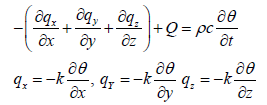Where, k is the thermal conductivity of media. Substitution of Fourier’s relation gives the following basic flow equation: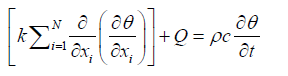The specified pressure, volume and ambient temperature areThe specified flowand the convection boundary conditions ison S3.

For coupled phenomena’s during interaction near the granular the contact with solid giveswhere σ is Stephan-Boltzmann constant, ε is the surface emission coefficient; α is the surface absorption coefficient and qr is coming flow per unit surface area. For transient problems it is necessary to specify a pressure field for a body at the time t=0; θ(x, y, z, 0)=θ0(x, y, z).The boundary conditions for the water wave problem are P=P0 on y=Given c>0, we are looking for periodic waves traveling at speed c. The profile η oscillates around the flat surface y=0 and the horizontal fluid velocity u is less than c at every point. For convenience we shall take the length scale to be 2π . Define the (relative) stream functionwithon the free surface, and letbe the vorticityAt least locally, away from a stagnation point (a point where u=c, v=d, w=0), ω is a function of Ψ . We will assume that there is a functionγ , called the vorticity function, such that ω =γ (ψ ) throughout the fluid. Thus, Δψ = −γ (ψ ). We define the relative mass flux as, which is independent of x by (2). Since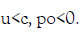Lethave minimum valueLetbe the closure of the open fluid domainGiven as set E with a smooth boundary, define for ∈N and α ∈ (0,1) the spaceof functionswith Holder continuous derivatives ( of index α) up to order m and of period 2π in the x, y and z variables. Our main results are as follows (Figure 2).Figure 2: Main results with the x, y and z variables.

#### Theorem 1

Let the wave speed c>0, the relative mass flow po<0 and an arbitrary α∈ (0,1) be given. Let y(s) be a C1+α function defined on [0,|po|] Such thatAndConsider traveling solution of speed c of the water wave problem (1)–(2) with vorticity function such that u<c throughout the fluid. There exists a connected set C of solutions (u,,,) in the Spaceappropriated to Sobolev Space for Nonlinear Partial Differential Equation, with the following properties.

1. C contains a trivial flow (with a flat surface η=0 );

There is a sequence of solutionsfor whichFurthermore, each solutionsatisfies.

1. It is easily thathave period 2π in axis of gravitation z;

2. Within each period the wave profile η has a single maximum (crest) at x=a, say, and a single minimum (through);

3. That u and η are symmetric while v is antisymmetric around the line x=a;

4., i.e., the profile of the wave is strictly decreasing from crest to trough.

#### Therom 2

(i) We make the same assumptions as in theorem 1, except we do not assume (4). Then there exists a connected set C with the same properties as in Theorem 1, except that 5 ii) is replaced by (B*) there is a sequence (Un) at either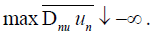#### Discussion

History of Stokes

In 1847 Stokes  studied irrotational periodic traveling water waves and some of their nonlinear approximations. The flat approach was developed Levi-civita  and Struik . Nevertheless in this work we construct a global continuum of such regular solutions with general vorticity. After that no other bifurcation point λ* can have this nodal pattern and the pattern persists all along C the nonlinear boundary condition. We reduce the alternative (a) that C is unbounded into the condition thatis unbounded in, then we prove that h is successively bounded inand finally ininHere we use the Schauder estimates and several basic a priori estimates of Lieberman and Trudinger  for nonlinear elliptic equation with nonlinear oblique boundary conditions.

#### Application

Thus alternatives are reduced to (a*) eitheris unbounded in L∞ (R), or (c) C contains in its closure a solution wherevanishes. Then we return to the original problem in the form of the Euler equation. Under assumption (4) we eliminate the last possibility (c) However, (a*) means that max, while (c) means that minfor some sequence of solutions.

Compacted granular

Granular matter has been the subject of numerous studies since two last decades [G1-G2] for two–dimensional granular system [G2] and some the propagation of two-dimensional [G3] inviscid gravity waves at the surface of a layer of water with a flat botton.

Many manipulations of granular by compaction to properties powders are well-known, but the manipulation the adhesion liquid onto compacted granular in four different scales: macroscopic scale, mesoscopic scale, microscopic scale and nanoscale is very complex and present the nonlinearity of behaviour waves water onto substrates solid of compacted granular throughout the porous. Interface liquid-solid generate by nonlinear dynamical system. The models developed can help to understand and make predictions about effects induced by multipara meters change such onto during flow liquid, stagnation and flow in porous of granular and amplitude of periodic stimulus. The multi-disciplinary team of scientists and support staff whose aim is to investigate the occurrence of scrapie in the water of world population and to provide advice on the control of penery of drinking water in the future. We do not understand if the complex of element‘s “Yakam Matrix” can in physical sciences; scientific understanding has been expressed in elegant theoretical constructs and has led to revolutionary technological innovation [8-11]. If the advances in understanding bifurcation behavior of liquid-solid interface of “Yakam Matrix” will follow the some trajectory of waves of water, granular compaction and dynamics of fluids at all scales, then we are still just at the beginning “Yakam Matrix”.

The study of networks pervades all of science, from fluids mechanics. The nonlinear dynamics: systems can often be modelled by differential equations dx/dt=v(x), where x(t)=(x1(t), …, Xn(t)) is a vector of state variables, t is time, and v(x)=(V1(x),…, Vn(x)) is a vector of functions that encode the dynamics.

#### Terminology and ConceptsWhereis the phase of the ith oscillator and i ω is its natural frequency, chosen at random from a lorentzian probability densityof width γ and mean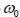. Using an ingenious self-consistency argument, Kuramoto solved for the order parameter that(a convenient measure of the extent of synchronization) in the limitandHe found thatWhere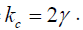In order words, the oscillation and stagnation of water through porous of granular are desynchronized completely until the coupling strength K exceeds a critical value kc . After that, the population splits into a partially synchronized three dimensional state.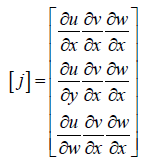#### Conclusion

The partials derivatives of x, y, z, in respect to u, v, w are found by differentiation of displacement of water through porous of granulars.We limit our investigation in Hilbert-Sobolev Spaces specified byFor our study“noyau” of operator ofin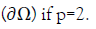#### References

1. Mukeba LK. Pinning study of contact line during droplet evaporation, PhD Dissertation, University of Liege, Belgium, 2007.
2. Johnson RS. A modern introduction to the mathematical theory of water waves, Cambridge University Press, UK, 1997.
3. Contanton A. Walter Strauss, Exact periodic traveling water waves with vorticity, CRAcad Sci Paris, Ser. 2002;1335:797-800.
4. Stokes G. On the theory of oscillatory waves, Trans. Cambridge Philos Soc. 1847;8:441-455.
5. Levi-Civita T. Determinazione rigorosa delle onde irrotazionali periodiche in acqua profonda. Rend Accad Lincei. 1924;33:141-150.
6. Struik D. DÃÆÃÂ©termination rigoureuse des ondes irrotationnelles pÃÆÃÂ©riodiques dans uncanal ÃÆÃÂ  profondeur finie. Math Ann. 1926;95:595-634.
7. Lieberman G, Trudinger N. Nonlinear oblique boundary value problems for nonlinear elliptic equations. Trans Amer Math Soc 1986;295:509546.
8. De Gennes PG. Granular matter: A tentative view. Rev Mod. Phys. 1999;71:S374.
9. Lumay G, Vandewalle N. Experimental study of granular compaction dynamics and different scales: grain mobility, hexagonal domains, and packing fraction. Physical Review Letters. PRL. 2005;95:028002.
10. Constantyin A. Walter Strauss, Exact periodic traveling water waves with vorticity, CRAS Paris, Ser I. 2002;335:797-800.
11. Boyer F, Fabrie P. Mathematical tools for the study of the incompressible NavierÃÂ¢Ãâ¬ÃâStokes equations and related models. Springer Series: Applied Mathematical Sciences. Springer, 2013.

Citation: Kabeya-Mukeba LY (2019) Interface Liquid-Solid: Global Bifurcation Multi-parameter of Flow Water Waves through Porous Compacted Granular by “Yakam Matrix”. J Appl Mech Eng. 8:321. doi: 10.35248/2168-9873.19.8.321

Copyright: © 2019 Kabeya-Mukeba LY. This is an open access article distributed under the term of the Creative Commons Attribution License, which permits unrestricted use, distribution, and reproduction in any medium, provided the original author and source are credited.